## Water Level Control in a Tank

This model shows how to implement a fuzzy inference system (FIS) in a Simulink® model.

This model controls the level of water in a tank using a fuzzy inference system implemented using a Fuzzy Logic Controller block. Open the `sltank` model.

```open_system('sltank') ```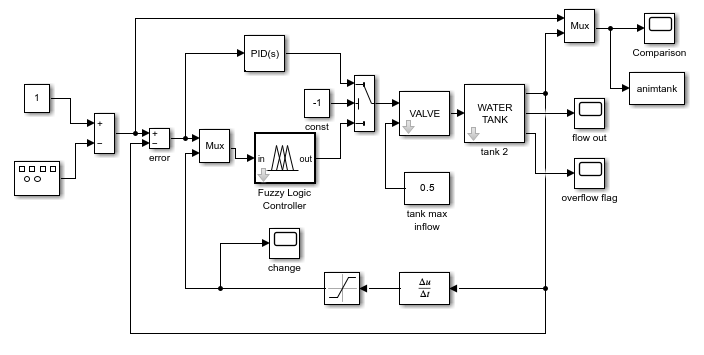For this system, you control the water that flows into the tank using a valve. The outflow rate depends on the diameter of the output pipe, which is constant, and the pressure in the tank, which varies with water level. Therefore, the system has nonlinear characteristics.

### Fuzzy Inference System

The fuzzy system is defined in a FIS object, `tank`, in the MATLAB® workspace. For more information on how to specify a FIS in a Fuzzy Logic Controller block, see Fuzzy Logic Controller.

The two inputs to the fuzzy system are the water level error, `level`, and the rate of change of the water level, `rate`. Each input has three membership functions.

```figure plotmf(tank,'input',1) figure plotmf(tank,'input',2) ```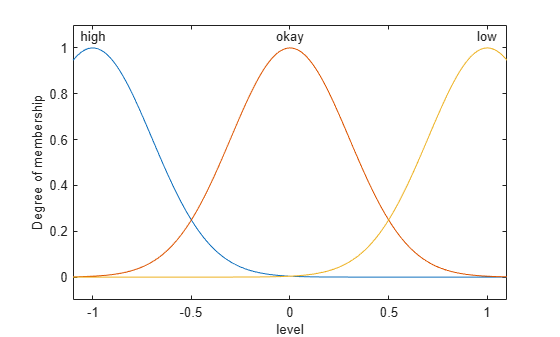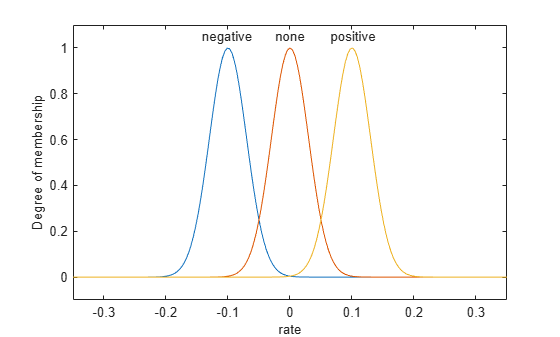The output of the fuzzy system is the rate at which the control valve is opening or closing, `valve`, which has five membership functions.

```plotmf(tank,'output',1) ```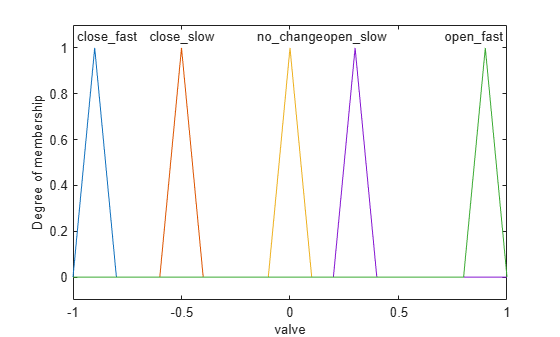Due to the diameter of the outflow pipe, the water tank in this system empties more slowly than it fills up. To compensate for this imbalance, the `close_slow` and `open_slow` valve membership functions are not symmetrical. A PID controller does not support such asymmetry.

The fuzzy system has five rules. The first three rules adjust the valve based on only the water level error.

• If the water level is okay, then do not adjust the valve.

• If the water level is low, then open the valve quickly.

• If the water level is high, then close the valve quickly.

The other two rules adjust the valve based on the rate of change of the water level when the water level is near the setpoint.

• If the water level is okay and increasing, then close the valve slowly.

• If the water level is okay and decreasing, then open the valve slowly.

```tank.Rules ```
```ans = 1x5 fisrule array with properties: Description Antecedent Consequent Weight Connection Details: Description ______________________________________________________ 1 "level==okay => valve=no_change (1)" 2 "level==low => valve=open_fast (1)" 3 "level==high => valve=close_fast (1)" 4 "level==okay & rate==positive => valve=close_slow (1)" 5 "level==okay & rate==negative => valve=open_slow (1)" ```

In this model, you can also control the water level using a PID controller. To switch to the PID controller, set the const block to a value greater than or equal to zero.

### Simulation

The model simulates the controller with periodic changes in the setpoint of the water level. Run the simulation.

```sim('sltank',100) open_system('sltank/Comparison') ```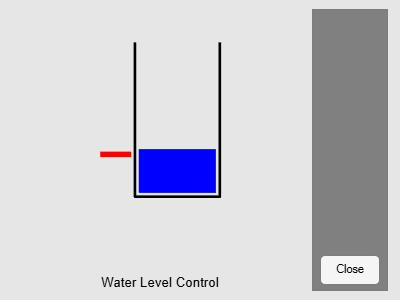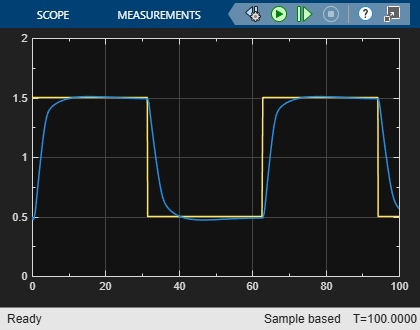The water level tracks the setpoint well. You can adjust the performance of the controller by modifying the rules of the `tank` FIS. For example, if you remove the last two rules, which are analogous to a derivative control action, the controller performs poorly, with large oscillations in the water level.